Understanding simple difinition contour lines

# Understanding simple difinition contour lines### Understand the simple definition contour lines on a topographic map to obtain and master land contour line

Level curves
1. Definition and terminology

The Contour lines are intended to give on a topographic map an overview of the real relief. A contour line ( fig. 10.1. ) is the intersection of the real relief with a horizontal plane of altitude given in round dimention (generally a whole number).
The curves are equidistant in altitude; their horizontal spacing depends on the slope of the terrain to be represented and on the scale of the plan or map. The terrain drawn flat on the map is visualized in three dimension. This is reinforced on the maps by coloring to underline the ridge lines: they represent the shadow created by a fictitious light whicjh would come from the northwest of the map.
On the example below, we can read on the plan view the slopes of the natural terrain; wy identify the summits, the topographic passes, the basins (or sinkholes), the breaks in slope.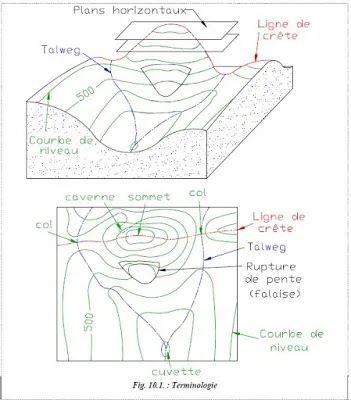1.1. Principe of interpolation

Understanding interpolation allows you to wisely choose the number and passion of the points to be surveyed.
The altitude at point M Located between the contour lines
530 and 540 is determined by considering the constantly sloping ground between A and B.
Points A and B are the closest points to M on contour lines 530 and 540; here ΔH = 10 m.

The slope at point M is : p = ΔH
ab
The distance ab is the real distance, that is to say the distance measured on the plan and divided by the scale of the plan.
The altitude of M is : HM = HA + am  ΔH
ab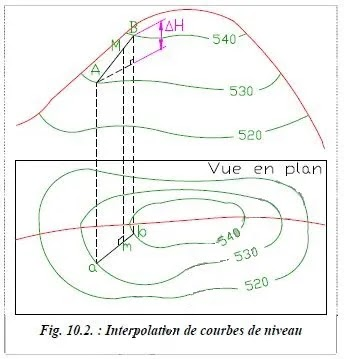This last formula can be applied with the distances measured on the plan; the scale factor simplifies.
1.2. Longitudinal plotting and cross sections
During a preliminary draft of the study of a road project, the designer needs a cross-sectional view of the natural terrain along the awis of the project he is studying: this graph is the cross sections. These two types of graphs make it possible to obtain, after several studies, an 'ideal' layout meeting the requirements of the project which are the cross sections. These two types of graphs make it possible to obtain, after several studies, an 'ideal' layout meeting the requirements of the project which are visibility, influence on the ground, maximum gradient, least cost, etc.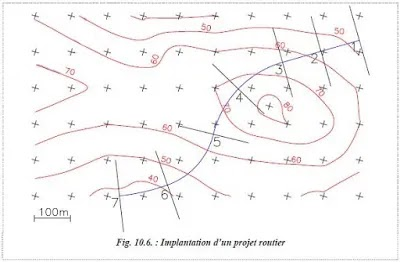2. Report of level curves

After carrying out the land survey, the work consists of reporting the data on paper. This tedious work is now supported by topography software, however it is instructive to have carried out at least one manual report to assimilate the method.
We consider 2 points surveyed on the ground 'a' and 'b' with respective altitudes Alta and Altb. The distance between these 2 points is L. The objective is to draw a  level curve by calculating the distance 'LaM'. The whole is  represented by the diagram.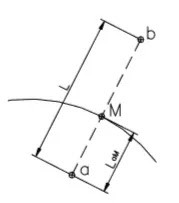The distance LaM is given by the relation:

Application 1  : The figure below represents the survey  of a pattern of points in order to draw the  level curve +129.50m
We identify the points that frame this curve: 17 and  19 are above, 18 , 20 and  24 are below. Points  31 and  23 are too far apart: they will not be taken into account. It remains to be determined by interpolation of the crossing points of the curve over the four  segments  1718 , 17 - 20 , 19 - 20 and  19 - 24 ; these segment are chosen so as to be as short and as perpendicular as possible to the futuretopographic contour line; for example, the segment  17 - 24 is discarded .3. Long and cross sections

3.1 Definitions and terminology

A longitudinal profile is the representation of a vertical section along the axis of a linear project (road, railway, pipeline, etc..).
The longitudinal profile is supplemented by cross sections which are vertical sections perpendicular to the axis of the project. Their establishment generally allows the calculation of earth movements ( cubatures ) and, for example, makes it possible to define the ideal layout of a project so as to equalize the volumes of excavated earth with the volumes of backfilled earth. (Not always easy!!)For example, in figure 10.10., a road project is shown as a centerline. The longitudinal profile constitutes a development along its axis on which the natural ground and the project are represented. The cross sections, regularly spaced, are a sectional view which provides the inscription of the road in the relief perpendicular to the axis.

3.2 Longitudinal sections

The longitudinal profile is a graph (fig. 10.11.) on which are plotted all the points of the natural ground and the axis of the project. It is established in the first place.
We rely on this document for the drawing of the cross section (fig. 10.12.).
Distances and   altitudes are given in meters to the nearest centimeter.

In general, a comparison plane is chosen with an altitude lower than the altitude of the lowest point of the project or of the natural terrain.
This comparison plane is the abscissa axis are plotted.

The scales of representation can be different in abscissa and in ordinates (in ratio of the order of 1/54 to 1/10) so as to emphasize the relief which may not appear on a project of great length..

We first draw the natural terrain ( TN ), generally in a black medium line.
Its layout is given by the position of each axis point of a cross section, the natural terrain being assumed to be straight between these point.
At the same time, information is reported in the box at the bottom of the graph: the horizontal distances between cross sections called partial distances, the cumulative distances (also called curvilinear abscissas) since the origin of the project and the altitude of each point.

The calculations of the positions of the characteristic points are reduced to straight-straight, straight-circle or staight-parabola intersections in the reference associated with the longitudinal profile.Fillings  ( in red ) and cattings  ( in blue ) can be colored differently.

The fictitious cross sections (zero surface) whose position must be determined (abscissa and possibly the altitude) are the points of intersection between the natural ground and the axis of the project; these particular profiles are useful for the calculation of cubatures.
It is necessary to know their position on the abscissa with respect to the two cross sections which frame them.

Drawing procedure:

1) Choice of the horizontal reference plane (Comparison plane)
2) Define the TN: layout + dimensions
3) Define project: layout + dimensions
4) Number the position of the cross sections
5) Indicate the distances (partial and cumulative)
6) Indicate the slope of the project
7) Indicate the geometric characteristics of the project: alignments and curves (plan view)

Example of a computerized longitudinal profile3.3 Cross sections

Cross  sections (cross-sections perpendicular to the project naxis) are used to calculate the following parameters:

₪  The position of the theoretical entry points of the earthworks
₪  The base of the project and its influence on the natural terrain
₪  Cubatures (volumes of cut and fill ).

The left lane must then be on the left side of the profile.
We start by drawing the natural terrain from a horizontal reference plane which is not necessarily that of the   longitudinal profile, so as to obtain the cross section at the maximum scale on the chosen format.

The scale of representation is of the order of 1/100 to 1/200  (up to 1/50 for the narrowest routes). There is no different scale in abscissa and in ordinate so as to be able to measure directly on the graph legths in all directions or surfaces

The abscissa of each point of the natural terrain (or of the project) is located in relation to the axis of the cross section (therefore negative on the laft and positive on the right), the ordinate is always the altitude of the point.
The standard template for the project is then superimposed on it (width of roadway, shoulders, ditches and embankment slopes ) from the axis point whose altitude has been determined on the longitudinal profile

This... .There are three types of cross sections (fig. 10.13.): embankment profiles , cuttings or mixed profiles .It should be noted that the presence of the ditch on these different types of profiles is only necessary in the event of the impossibility of natural water flow. For example, compare the fill profile and the mixed profile .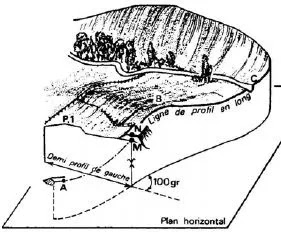Cross section principle
Example of a Computerized Cross Section